| my account | login-logout | resources | classroom help | support | catalog | home | get webcard |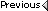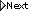» Online Classroom   »   » Public Discussion of Cel Nav   » Hawaii by Sextant,Problem 11

Author Topic: Hawaii by Sextant,Problem 11
 climber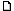posted July 23, 2016 02:17 PM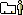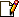For this problem the sun declination, as looked up in the NA table in the book, for July 12 at 21:45:57 was N21 degrees 55.6 minutes. For the next sight at 23:45:20 it was N21 degrees 54.9 min. Both of these values are from the NA tables in the book.I worked both sights using the declination degrees in Box 4 as N22 degrees because both table values were much closer to 22 degrees than 21 degrees. Using the 22 degree value and the appropriate LHAs and a-Lats my plotted fix came out way NorthWest of the DR position.I looked at the book and saw that the declination degrees in box 4 of the answer sheet was N21 degrees. When I went back and worked the problem using the 21 degree value I came out within a mile of the book answer. I don't understand how this difference can cause such an error in my plotting. Without the plot I don't know if you can answer that question. I can send photos of the worksheets if necessary.Secondly, why was the declination value chosen as N21 degrees in the book when both declinations from the book tables were less than 5 min. less than 22 degrees? A value of N22 degrees would make more sense using the usual rule of arithmetic for rounding.I can send photos of my worksheets after I replot them in ink so they are legible. I've worked this problem several times so I must be making the same mistake each time. I can obtain the correct answer using the N21 degree value for the sun's declination but not using the N22 degree value.Thank you. From: apalachicola
 Capt Steve Millerposted July 23, 2016 03:22 PMWe do not round the Declination as you outline in your question. We use the degrees portion to find the data required from the Sight Reduction Tables. Then we use the 'd' found in the SR Tables along with the minutes portion of the Declination to calculate the minutes correction.Your method would change the Table Hc, the 'd' and the Z from the correct values when using the correct Declination degrees without rounding it. From: Starpath
 climberposted July 24, 2016 01:47 PMI s what you mean in that it works and I can work the problem. You use the declination degree value from the NA and disregard the minutes. I don't remember this from the CN textbook. Can you point out where this is as I can't find it.I'm not sure I understand the reasoning however. The declination in the NA is the altitude of the sun if you took a sight from the equator at the whole-hour time. The Sight Reduction Tables give the altitude of the sun at your assumed Latitude for your LHA when the sun is at the declination given in the NA. If you don't do any rounding the d-corr. Is irrelevant. . If that's the case and the declination from an equator sight is 21 degrees 59 minutes I don't understand why you wouldn't round up to 22 degrees for using the SR Tables. Does this have to do with the way the SR Tables are constructed? Thank you. From: apalachicola
 Capt Steve Millerposted July 24, 2016 04:02 PMThere is an example of the Declination being added to Box 4 on page 62 of the Cel Nav textbook.The Declination is changing by the second so it is Not irrelevant. The actual Declination is determined by looking at the Daily Pages for the Hourly value and the Increments and Corrections for the minute correction.In the Problem 11 in HBS the Declination is N21° 54.6' for the 1645 sight not N21° 59' as you keep indicating in your posting.I indicated in my last post that if you rounded the Declination to N22° the Hc, d, & Z would be different from the N21° values. N22° values are Hc = 53° 47.3, d =27.6, Z = 99.0where the values forN21° values are Hc = 53° 18.6, d =28.7, Z = 100.5The final results for the N21° calculation are Hc = 53° 34.7 and Zn =259.5°.The final results for the N22° calculation are Hc = 53° 47.3 and Zn =261°. This is assuming that you rounded the Declination to N22° 0'.That is a big difference in the results -- Hc difference of 28.7'. Or when you calculate the intercept w/N21 a= 36.9A miles @ 259.5° and w/N22 a= 39.5A miles @ 261° From: Starpath

 All times are Pacific